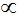## Gaseous State

Only 11 elements ( H, O, N, F, Cl, He ,Ne, Ar, Kr, Xe, Rn exists in gaseous state under normal conditions.

#### Characteristic Physical Properties Of Gases

·       Gases are highly compressible.
·       Gases exert the equal pressure in all direction.
·       As compared to solid and liquids, gases have much lower density.
·       Gases don’t have definite (fix) shape and volume.
·       Gases mix completely and evenly in all proportions.

## Gas Laws

### Boyle’s law:

It is also known as Pressure Volume relationship.
As per Boyle’s law, ”At constant temperature and fixed amount of gases in no. of moles, its pressure varies inversely with its volume.”
Mathematically, at constant T and n,
P1/V ……………..1
P = k1 x 1/V   =   k1/V ………..2
Where, P = Pressure, V = Volume and k = proportionality constant and value of k1 depends upon Pressure P and Volume V.
Also,    K1 = PV ………..3
According to above relation, product of pressure P and volume V remains constant, if we fixed the amount of gas at constant temperature. You read these first class chemistry notes for classes 11 at online classes by www.ChemistryNotesInfo.com.
So,  P1V1 = P2V2 = Constant  ………4
Then,   P1/ P2 = V2/V1   ……………..5
As we know, Density is equal to mass divided by volume i.e. d=m/V
So, V = m/d …………6
From equation 2 & 6,
P = k1d/m
·       d = (m/k1)/P = k’P ……………7
Where, k’ = m/k1

### Charle’s Law:

This is also known as Temperature and Volume relationship.
As per Charle’s law, “At constant pressure and fixed mass of gas, Volume is directly proportional to Absolute temperature.”
Mathematically, at constant P and n,
VT
Also, V=k2T
Where, K2 is a constant.

### Thermodynamic Scale:

Kelvin scale of the Temperature is known as the Thermodynamic Scale which is utilized in many scientific works.

### Kelvin Scale:

To obtain temperature in Kelvin scale we add 273.15 (generally 273) in Celsius temperature.
i.e. K= 273.15 + °C (degree  Celsius)

### Gay Lussac’s Law:

This law is also known as Pressure and Temperature relationship.
According to Gay Lussac’s Law “At constant Volume and fixed amount of gas, pressure is directly proportional to temperature.”
Mathematically, at constant V and n,
PT
Also, P=k3T
Where, K3 is a constant.

This law is also known as Volume and amount relationship.
According to Avogadro’s law “Equal volume of all the gases under same condition of pressure and Temperature contain equal no. of molecules.”
Vn ………….1
V=k4n ……………2
Where, V is volume, k4 is a constant and n is no. of moles of gas.

One mole has 6.022x1023 no. of molecules which is called as Avogadro’s constant. As we know, mole is equal to mass divided by molar mass.
So n = m/M  ……………..3
Then from equation 2 & 3
V = k4(m/M)
M = k4. m/V
M = k4.d    {here . represents multiplication}
Where M is molar mass, m is mass and V is volume, K4 is constant and d is density.